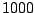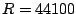Next: Audio and control computations Up: Wavetables and samplers Previous: Automatic read point precession   Contents   Index

Exercises

1. If a wavetable withsamples is played back at unit transposition, at a sample rate of 44100 Hertz, how long does the resulting sound last?

2. A one-second wavetable is played back in 0.5 seconds. By what interval is the sound transposed?

3. Still assuming a one-second wavetable, if we play it back periodically (in a loop), at how many Hertz should we loop the wavetable to transpose the original sound upward one half-step?

4. We wish to play a wavetable (recorded at), looping ten times per second, so that the original sound stored in the wavetable is transposed up a perfect fifth (see Page). How large a segment of the wavetable, in samples, should be played back?

5. Suppose you wish to use waveform stretching on a wavetable that holds a periodic waveform of period 100. You wish to hear the untransposed spectrum at a period of 200 samples. By what duty factor should you squeeze the waveform?

6. The first half of a wavetable contains a cycle of a sinusoid of peak amplitude one. The second half contains zeros. What is the strength of the second partial of the wavetable?

7. A sinusoid is stored in a wavetable with period 4 so that the first four elements are 0, 1, 0, and -1, corresponding to indices 0, 1, 2, and 3. What value do we get for an input of 1.5: (a) using 2-point interpolation? (b) using 4-point interpolation? (c) What's the value of the original sinusoid there?

8. If a wavetable's contents all fall between -1 and 1 in value, what is the range of possible outputs of wavetable lookup using 4-point interpolation?Next: Audio and control computations Up: Wavetables and samplers Previous: Automatic read point precession   Contents   Index
Miller Puckette 2006-12-30